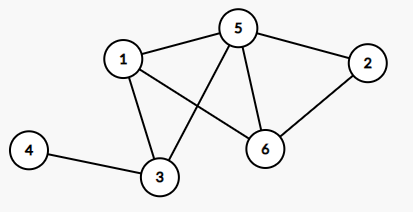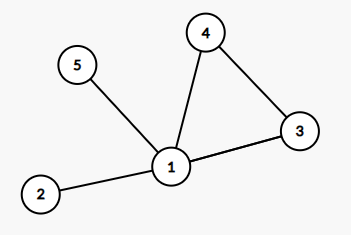# Graph NotationThere are different types of graph notations. Here I’d like to present a nice and compact way in the form of a Vertex Lists and Edge Lists, which I came across during my studies at the MSE in Zurich.

[Note: All graph images shown in this post have been taken from graphical representations of graphs designed using the cs-academy’s graph editor. Thanks a lot for this great tool!]

For the purpose of demonstration, let’s assume we want to have a data-representation of the following graph:A Graph we want to find a notation for

#### Vertex List

The Vertex List is a list which gives the neigbor vertices for each vertices in the graph. If we would like to give an algorithm to generate a Vertex List, it would probably look as follows:

``````print # of vertices
print # of edges
foreach i in vertices
print # of neighbor vertices of node i
foreach j in neighbor_vertices(i)
print node j``````

So let’s apply the algorithm and try, if we can determine how the upper graph’s Vertex List looks like:

``[5,5,4,2,3,4,5,1,1,2,1,4,2,1,3,1,1]``

Now let’s do it step by step: The list starts with a 5, indicating that we have 5 vertices (1,2,3,4 and 5), the second 5 stands for the number of edges, which is 5 as well. We get:

``[5,5,``

Now let’s loop through all vertices in ascending order and for each one first print the number of neighbor-nodes, the node is connected to, and then list all these neighbors’ node IDs in ascending order: Node 1 has 4 neighbor nodes, being 2,3,4,5. Note, that we do not mention the node under consideration, so there is no 1 to be printed here. Thus we get:

[5,5,4,2,3,4,5,

Now, we do the same for node 2. It has only 1 neighbor node, being 1:

``[5,5,4,2,3,4,5,1,1,``

Now we just repeat the same steps for the other nodes left, Nodes 3,4 and 5. We then get the uniquely defined solution shown above:

``[5,5,4,2,3,4,5,1,1,2,1,4,2,1,3,1,1]``

#### Edge List

The Edge List is a list which gives the edges for each vertices in the graph by telling the nodes, the edges connect. If we would like to give an algorithm to generate a Edge List, it would probably look as follows:

``````print # of vertices
print # of edges
foreach i in vertices

foreach j in neighbor_vertices(i)
print node i
print node j``````

So let’s apply the algorithm and try, if we can determine how the upper graph’s Edge List looks like:

``[5,5,1,2,1,3,1,4,1,5,2,1,3,1,3,4,4,1,4,3,5,1]``

Now let’s do it step by step: The list starts with a 5, indicating that we have 5 vertices (1,2,3,4 and 5), the second 5 stands for the number of edges, which is 5 as well. We get:

``[5,5,``

Now let’s loop through all vertices in ascending order and for each one first print the id of the node considered, then the id of the neighbor node, both in ascending order: Node 1 has 4 neighbor nodes, being 2,3,4,5. In contrary to the Vertex List, here we do mention the node under consideration for each edge, we want to report. Thus after reporting the edges of node 1 we get:

``[5,5,1,2,1,3,1,4,1,5,``

Now we want to report the edges of node 2. Since from node 2 we can only go to node 1, we get:

``[5,5,1,2,1,3,1,4,1,5,2,1``

Next we report the edges of node 3. From node 3 we can go to node 1 and 4, thus we get:

``[5,5,1,2,1,3,1,4,1,5,2,1,3,1,3,4``

Now we just repeat the same steps for the left nodes 4 and 5, ending up with the solution provided above:

``[5,5,1,2,1,3,1,4,1,5,2,1,3,1,3,4,4,1,4,3,5,1]``

Note, that these notations are somewhat redundant. Of course, in undirected graphs we could have a more compact notation when preventing to mention connections twice in both directions. However, here we have the advantage that this approach can easily be extended for directed graphs.

Categories: